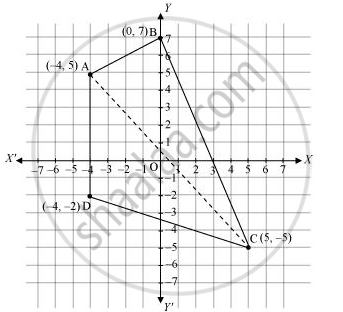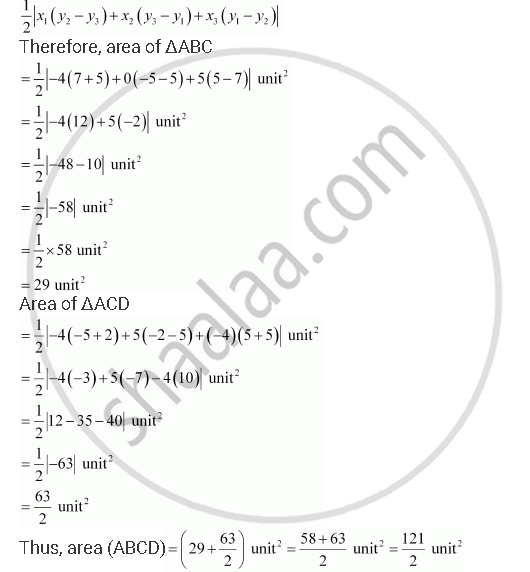CBSE (Arts) Class 11CBSE
Share

# Draw a Quadrilateral in the Cartesian Plane, Whose Vertices Are (–4, 5), (0, 7), (5, –5) and (–4, –2). Also, Find Its Area. - CBSE (Arts) Class 11 - Mathematics

#### Question

Draw a quadrilateral in the Cartesian plane, whose vertices are (–4, 5), (0, 7), (5, –5) and (–4, –2). Also, find its area.

#### Solution

Let ABCD be the given quadrilateral with vertices A (–4, 5), B (0, 7), C (5, –5), and D (–4, –2).

Then, by plotting A, B, C, and D on the Cartesian plane and joining AB, BC, CD, and DA, the given quadrilateral can be drawn asTo find the area of quadrilateral ABCD, we draw one diagonal, say AC.

Accordingly, area (ABCD) = area (ΔABC) + area (ΔACD)

We know that the area of a triangle whose vertices are (x1y1), (x2y2), and (x3y3) isIs there an error in this question or solution?

#### Video TutorialsVIEW ALL 

Solution Draw a Quadrilateral in the Cartesian Plane, Whose Vertices Are (–4, 5), (0, 7), (5, –5) and (–4, –2). Also, Find Its Area. Concept: Slope of a Line.
S